## Et-Function

A function which arises in Fractional Calculus.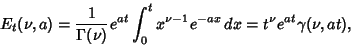(1)

where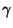is the incomplete Gamma Function and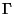the complete Gamma Function. The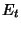function satisfies the Recurrence Relation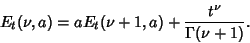(2)

A special value is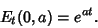(3)

Abramowitz, M. and Stegun, C. A. (Eds.). Exponential Integral and Related Functions.'' Ch. 5 in Handbook of Mathematical Functions with Formulas, Graphs, and Mathematical Tables, 9th printing. New York: Dover, pp. 227-233, 1972.